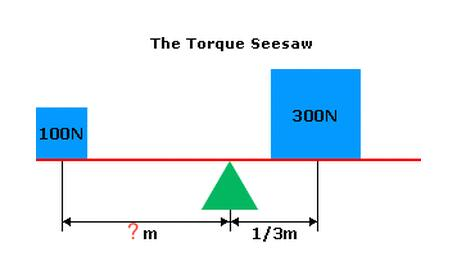Torque Sensor
You are here: » » Torque Sensor

## Torque Sensor

TM is a China torque sensor manufacturer

Torque is a twisting force, generally that causes something to rotate around an axis or other point. The force that causes a wheel to rotate around an axle is an example of torque. The tendency of a force applied to an object to make it rotate about an axis.From torque seesaw above, you could easily learn how torque works.

Torque= D(Distance) X M(Mass), by this formula, we can figure out unknown distance above, because this seesaw is balanced, we can set both D X M equal to each other.

So 100N X ?m = 300N X 1/3m.

Multiplying that out, you get 100N X ?M = 100N.m.  Therefore the unknown distance must equal 1 meter.

Besides, the torque on left side or right side is 100N.m.

The unit for torque is the newton meter (N.m) or foot pounds (ft.lb).

Reference:

torque of the town

## PRODUCT CATEGORY

Product Inquire
TM Sensor Co., Ltd.（Sensor BU）
TM Automation Instruments Co., Ltd.